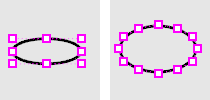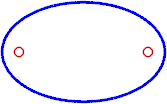# EllipseCurve

Ellipse >

<Options>

The Ellipse command draws a closed elliptical curve.

#### Steps

1. Pick the center.
2. Pick the end of the first axis.
3. Pick the end of second axis.
Command-line options
##### Default

Creates the ellipse from the center, first axis, and second axis.

#### Default steps

1. Pick the center.
2. Pick the end of the first axis.
3. Pick the end of second axis.

##### Deformable

A NURBS approximation of the curve will be created with the degree and number of points you specify.Not deformable degree 2 (left); Deformable degree 3 (right).
##### PointCount

Sets the number of control points in the curve.

Vertical

Draws the ellipse center point and axes perpendicular to the construction plane.

#### Vertical steps

1. Pick the center.
2. Pick end of the first axis.
3. Pick the end of the second axis.

Corner

Draws the ellipse from the corners of an enclosing rectangle.

#### Corner steps

1. Pick a corner for the enclosing rectangle.
2. Pick the opposite corner of the enclosing rectangle.

Diameter

Draws the ellipse from points on its axes.

#### Diameter steps

1. Pick the start of the first axis.
2. Pick the end of the first axis.
3. Pick the end of the second axis.
##### FromFoci

Draws the ellipse from focus points and a point on the curve.

#### FromFoci steps

1. Pick the first focus.
2. Pick the second focus.
3. Pick a point on the ellipse curve.
##### MarkFoci

Places a point object at the focus locations.AroundCurve

Draws the ellipse around a curve.

#### Steps

1. Pick the center on a curve.
2. Pick the end of the first axis.
3. Pick the end of the second axis.